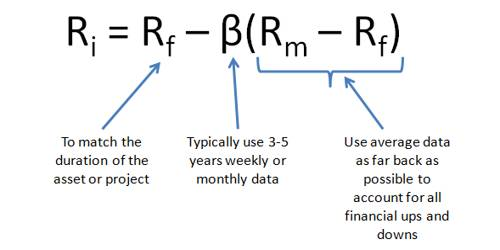# Differentiate between Arbitrage Pricing and Capital Asset Pricing Theory

The capital asset pricing model (CAPM) provides a formula that calculates the expected return on a security based on its level of risk. The formula for the capital asset pricing model is the risk-free rate plus beta times the difference of the return on the market and the risk-free rate. Arbitrage pricing theory (APT) is a well-known method of estimating the price of an asset. The theory assumes an asset’s return is dependent on various macroeconomic, market and security-specific factors.

Differentiate between Arbitrage Pricing Theory (APT) and Capital Asset Pricing Theory –

The context of differentiation is given below:

CAPM

1. It means the capital asset pricing model.
2. CAPM is based on an investor’s portfolio demand and equilibrium arguments.
3. It is based on risk-return trade-off.
4. It is difficult to find a good proxy for market return.
5. It has a simple beta.
6. CAPM is a single factor model.
7. CAPM requires that the market portfolio be efficient.
8. CAPM assumes that the probability distributes of asset returns are normally distributed.

APT

1. It means arbitrage pricing theory.
2. APT is based on the factors model of returns and the approximate arbitrage arguments.
3. It is based on mathematical and statistical data theory.
4. It is difficult to identify approximate factors.
5. It has several relevant data.
6. APT is a multifactor model.
7. There is no special role in the market portfolio in APT.
8. APT does not make any assumption about the distribution of asset returns.

These are the context of differentiation between CAPM and APT.